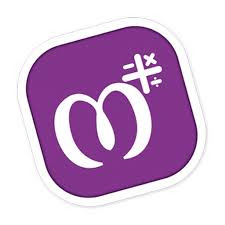## Saturday, May 9, 2020

### Download MAthry A way of Learning Math Supper Application from here

Speed up mathematical calculations with Mathry!

A Simple yet amazing math application for students, teachers and parents.

Increase your brainpower using thousands of mathematical questions/quiz.Excellent for preschool and grade school kids to learn basic arithmetic ideas like Addition, Subtraction, Multiplication, Division, Decimals, Fractions, pure mathematics so on.Learn these basic operations:
✓ Subtraction
✓ Multiplication
✓ Division

Other Features:
☆ Worksheet Generator (Teachers or Parents can use for an exam)
☆ Basic Operations on Number Base
☆ Fractions and Decimals
☆ Mixed Operators
☆ Percentage
☆ Square
☆ Square Root
☆ Cube
☆ Cube Root
☆ Find Missing
☆ and Much More!

Languages available:
» English
» Spanish
» Hindi
» Gujarati